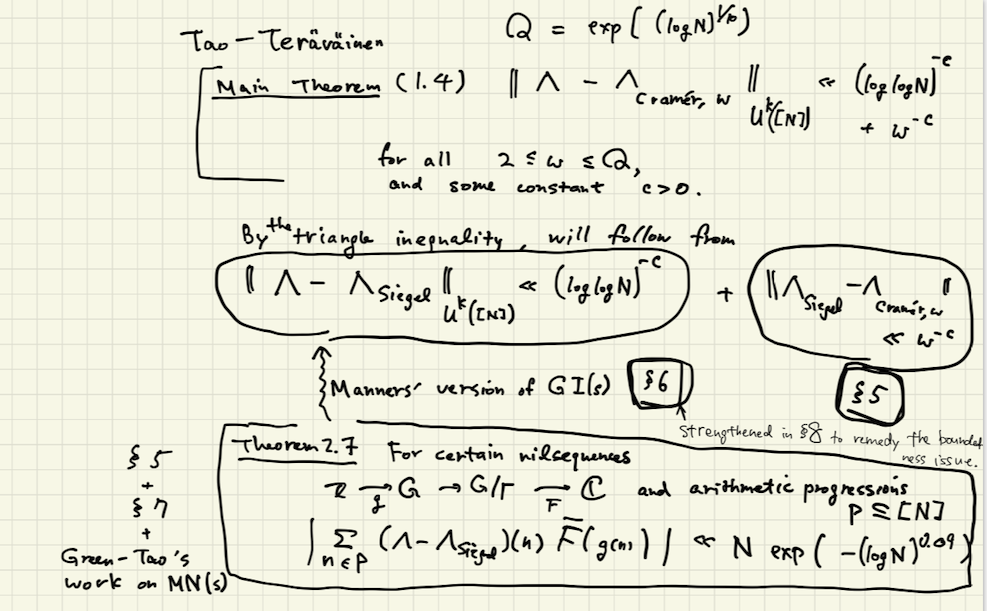[tex: ]

# Seminar program - season 2

We are following the Tao-Teräväinen paper: https://arxiv.org/abs/2107.02158 .

Tao's blog post about the above paper might also help: https://terrytao.wordpress.com/2021/07/05/

Part III

### Motivation

Write $Q:= \exp ( (\log N)^{1/10} )$.

Note that Q defined in this way grows slower than any positive power of $N$ because $N^{c} = \exp (c \log N)$ and $c\log N > (\log N )^{1/10}$. It grows faster than any power of $\log N$ because $\log ^A N := (\log N)^A = \exp (\log ( \log ^A N ) ) = \exp ( (\log \log N ) + \log A )$ and we know $(\log N )^{1/10 } > \log \log N$.

The main result is the bound of the following Gowers uniformity norm, with some sufficiently small $c>0$:

$\| \Lambda - \Lambda _{Cramér,Q} \| _{U^k ([N])} \ll (\log \log N )^{-c} +Q^{-c} .$

Let's write $\| - \| := \| - \| _{U^k([N])}$ to make it easier to type.

By the triangle inequality of $\| - \| _{U^k}$, this would follow from:

$\| \Lambda - \Lambda _{Siegel} \| \ll (\log \log N)^{-c} \quad \text{and }\quad \| \Lambda _{Siegel} - \Lambda _{Cramér,Q} \| \ll Q^{-c} .$

The latter inequality is easier and proved in §5.

The former requires substantial work.

By Manners' form of GI(s) explained in §6, this will follow from Theorem 2.7:

for certain nilsequences $\mathbb Z \xrightarrow{g} G\to G/\Gamma \xrightarrow{F}\mathbb C$ and arithmetic progressions $P\subset [N]$, we have

$\sum _{n\in P} (\Lambda - \Lambda _{Siegel})(n) \overline F (g(n) ) \ll N\exp ( (-\log N)^{0.09 } ) .$

This estimate is the main part of the paper. The proof requires §5 + §7 + former Green-Tao work on MN(s).By the way, §1 of the paper is introduction, §2 is an extended introduction including def of $\Lambda _{Siegel}$, §3 is a one-page section about the notation, §4 is recollection of $\| - \| _{U^k([N])}$.

The main content spans through §§5-8 (as we will see below). §9 explains the application to linear equation in primes. The final section §10 explains another application.

### Seminar Program - Part I

The following topics can be studied independently, so let's distribute them and give lectures to each other:

#### Basics of $\Lambda _{Cramér,w}$

Cover §5.1. The computation is annoying. But eventually we'll have to be comfortable with this type of computation.

#### Basics of $L (s,\chi )$ and Siegel zeros

What is $L(s,\chi )$, to begin with? (Read §5.9 of the Iwaniec-Kowalski book, say.)

What are the (Landau-)Siegel zeros? (Read p.106 of the Iwaniec-Kowalski book, say.)

Theorem 2.7 (p.122) of that book (which should motivate $\Lambda _{Siegel}$).

Wikipedia would help too. https://en.wikipedia.org/wiki/Siegel_zero

#### Manners' GI(s)

State Theorem 6.2 of Tao-Teräväinen. To do so, you will have to recall relevant notions for the audience. (I believe all the relevant notions can be found within the paper.)

[optional] Explain the implication (Theorem 2.7 of Tao-Teräväinen) $\Rightarrow$ $\| \Lambda - \Lambda _{Siegel} \|_{U^k([N])} \ll (\log \log N )^{-c}$, perhaps ignoring the "boundedness" issue for now.

[optional but preferred] What would be Manners' GI(s) for $\mathbb Z^n$ if we implement this blog post of Tao? https://terrytao.wordpress.com/2015/07/24/

### Part II

Having gone through Part I, we will be ready to study the following topics. Again, they can be studied independently of each other.

#### Toward the conparison of $\Lambda _{Siegel}$ and $\Lambda _{Cramér,Q}$

Present the first half of §5.2 (p. 20 -- first quarter of p. 22).

This seems to depend on what we have learned about Siegel zeros in Part I.

#### The first 1/3 of §7

Here, they prove Prop. 2.2, the main theorem with the test function $F$ constant.

We want to understand this part in depth.

#### Manners' GI(s) continued

Read §8, where they modify Manners' GI(s) so that it can be applied to non-bounded functions like $\Lambda - \Lambda _{Siegel}$.